Function Repository Resource:

Return the radical of an integer, the product of its distinct prime factors

Contributed by: Ed Pegg Jr

## Details and Options

Integer mathematical function, suitable for both symbolic and numerical manipulation.
The integer radical is also known as the squarefree kernel or largest squarefree divisor.
ResourceFunction["IntegerRadical"] gives the product of the distinct prime factors.
For a number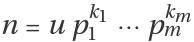with u a unit and pi primes, ResourceFunction["IntegerRadical"][n] returns p1pm.
ResourceFunction["IntegerRadical"][m+I n] automatically works over Gaussian integers.

## Examples

### Basic Examples (1)

The product of distinct prime factors of a few numbers:

 In:=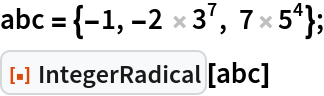Out=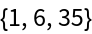### Scope (2)

Some examples of a+b=c:

 In:=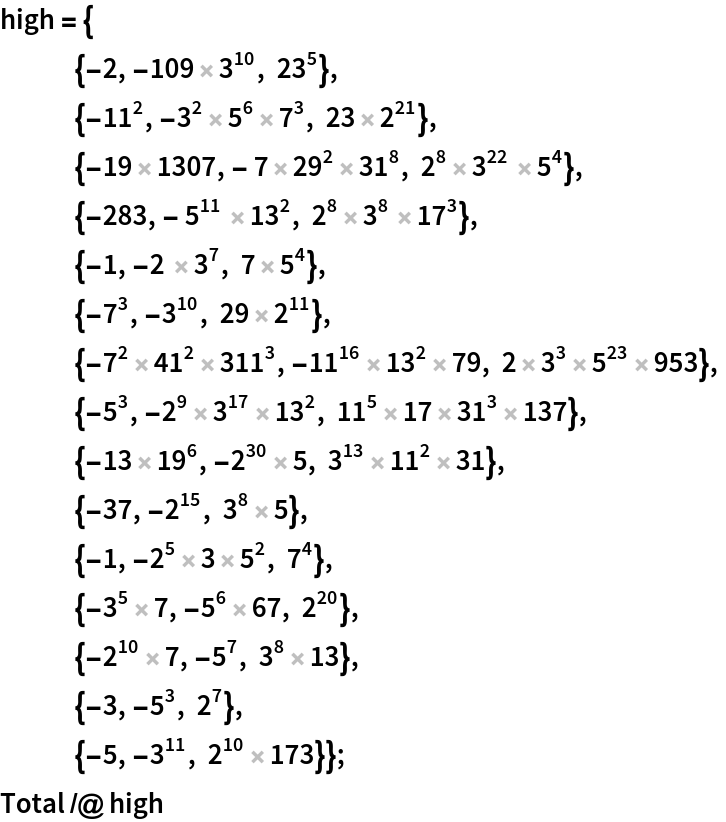Out=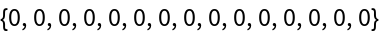Quality values for these examples of the abc conjecture:

 In:=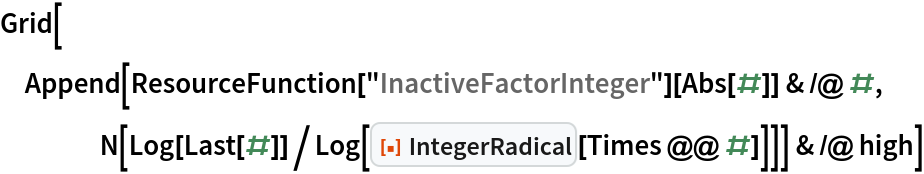Out=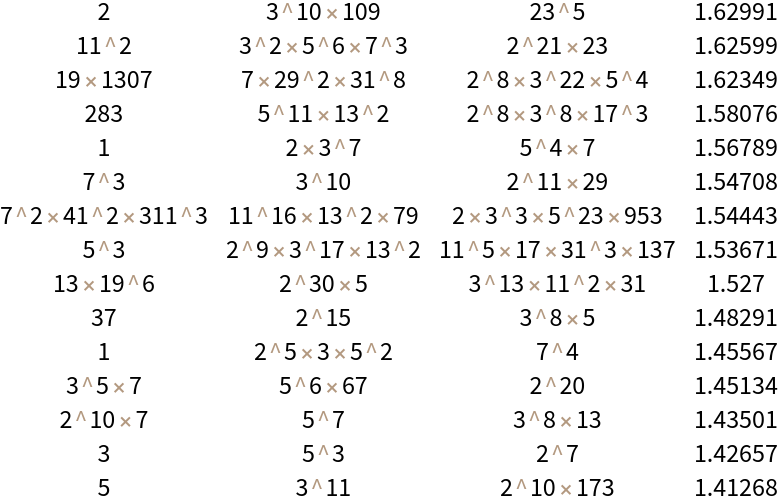Gaussian integers are supported:

 In:=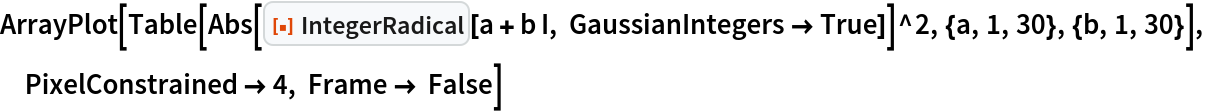Out=## Version History

• 1.0.0 – 01 March 2021